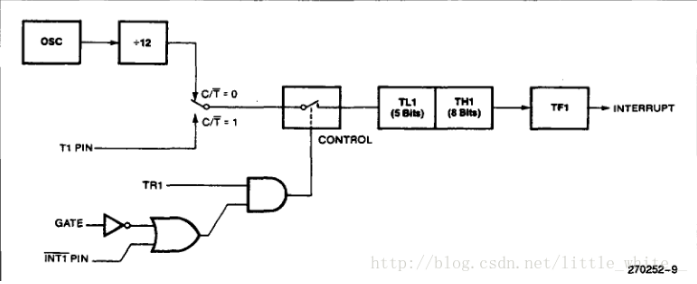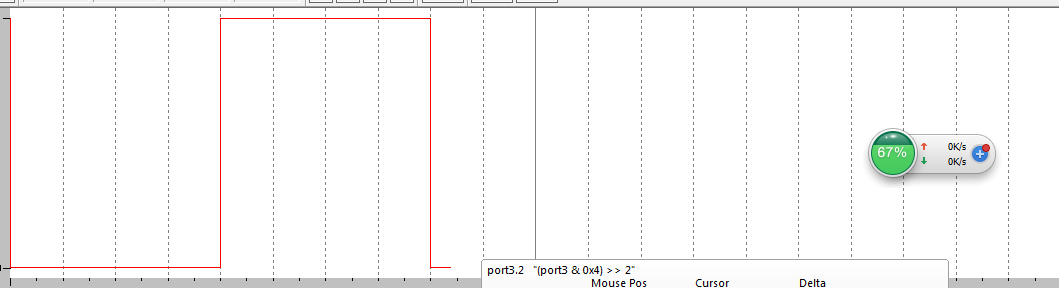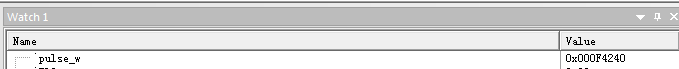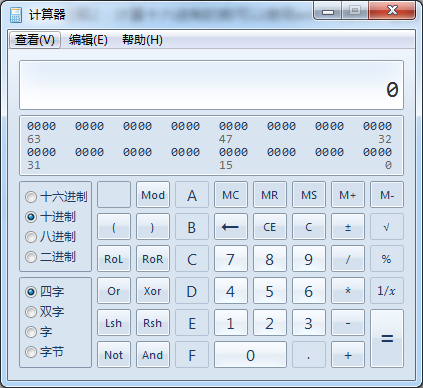## 51单片机一种精确的判断脉冲宽度的方法_单片机测脉冲宽度-程序员宅基地``````#include <reg51.h>
#include <intrins.h>

#define uint unsigned int
#define uLint unsigned long int		//长整型

uLint pulse_w = 0 ;//计算脉冲的时间，用长整型可以达到10的9次方us，如果用uint，最大只能达到65535us（还不到100ms）
sbit in = P3^2 ;

void Int0 (void) interrupt 0
{
pulse_w += TL0 ;
TL0 = 0 ;
}

void Time0(void) interrupt 1
{
pulse_w += 256 ;//计数寄存器溢出，直接加最大值
}

int main()
{
//初始化
TMOD = 0xA ; //定时器0，模式2，GATE0 = 1
TH0 = 0 ;    //填初值
TL0 = 0 ;
TR0 = 0 ;
ET0 = 1 ;//开定时器0中断

IT0 = 1 ;//外部中断0下降沿触发中断
EX0 = 1 ;//开外部中断0
EA = 1 ;//开总中断

while(1)
{
if(in == 0)//见下面的解释
TR0 = 1 ;

}
}
``````

``````
signal void test(double cc)
{
port3 &= ~(0x1<<2)  ;
swatch(1) ;
port3 |= (0x1<<2) ;
swatch(cc) ;
port3 &= ~(0x1<<2) ;
swatch(0.1) ;

_break_ = 1 ;

}
``````